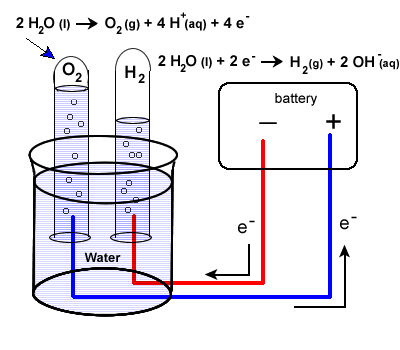Chemistry 30

Redox Reactions: Module Index | Practice Problems | Assignments | Student Lab | Research Ideas | Teacher ResourcesRedox Reactions & Electrochemistry

3.3 Electrolysis of Water

Our second example of electrolysis and electrolytic cells involves the breakdown of water. We will find a situation very similar to the electrolysis of molten NaCl.

The following equation represents the breaking apart of H2O(l):

2H2O(l) → 2H2(g) + O2 (g)

It may be more difficult to predict the half-reactions involved, but they are:

 E° reduction 2H2O(l) + 2e- → H2 + 2 OH- -0.83 V oxidation 2H2O(l) → O2 + 4H+ + 4e- -1.23V (see note below for net equation) net voltage required - 2.06V

The set-up will be very similar to our last example with some minor differences. Water does not carry a charge well, so an electrolyte is added to the water. Vinegar, a weak acid (acetic acid) may be used. To collect the hydrogen and oxygen gases produced, inverted test tubes are often added, as shown in our diagram below.

Again, take special care to trace the path of the electrons. Unless electrons make a complete circuit, a reaction will not occur.

1. Electrons are "produced" in the battery at the anode, the site of oxidation.

2. The electrons leave the electrochemical cell through the external circuit.

3. These negative electrons create a negative electrode in the electrolytic cell which causes the reduction of water.

Note that the area around this electrode will become basic as OH- ions are produced.

2H2O(l) + 2e- → H2(g) + 2 OH-(aq)

1. Meanwhile the the positive electrode water will undergo oxidation:

2H2O(l) → O2 (g) + 4H+ (aq) + 4e-

1. Electrons produced during this oxidation process will return to the electrochemical cell.Scavenger Hunt A Hoffman Apparatus and a Brownlee Apparatus are two different pieces of equipment commonly used to carry out the electrolysis of water. Locate pictures of them.

A note about the balanced equation for the electrolysis of water:

You may notice from the half reactions that adding up the equations doesn't initially give us our net equation of

2H2O(l) → 2H2(g) + O2 (g)

Once you balance for electrons (multiply the reduction equation by 2) you'll find the equations actually add up to :

6H2O(l) → 2H2(g) + O2 (g) + 4H+ (aq) + 4 OH-(aq)

The hydrogen and hydroxide ions will combine to form 4 moles of H2O(l). Finding our net amount of H2O(l) involved gives us our final equation:

2H2O(l) → 2H2(g) + O2 (g)